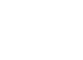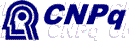WoLLIC'2000
7th Workshop on Logic, Language, Information and Computation

August 15-18, 2000

Interest Group in Pure and Applied Logics (IGPL)
European Association for Logic, Language and Information (FoLLI)
Association for Symbolic Logic (ASL)

Funding,Fundação Norte-Rio-Grandense de Pesquisa e Cultura (FUNPEC)
Pró-Reitoria de Extensão, Univ. Fed. do Rio Grande do Norte (PROEX-UFRN)

Organisation
Centro de Informática, Universidade Federal de Pernambuco (CIn-UFPE
Departamento de Informática e Matemática Aplicada, Univ. Fed. do Rio Grande do Norte (DIMAP-UFRN)

### Title and Abstract of Tutorial Lectures

The Optimal Implementation of functional programming languages
by Andrea Asperti (Department of Computer Science, University of Bologna, Italy)

In foundational research of almost thirty years ago, Jean-Jacques Lévy attempted to characterize formally what an optimally efficient reduction strategy for the lambda-calculus would look like, in the sense of avoiding useless duplication of work along the reduction.

In elaborating his notion of optimal reduction, Lévy introduced a labelled variant of lambda-calculus where all subterms of an expression are annotated with labels that code the history of a computation. He proposed the idea of a "redex family" - redexes in a term with identical labels - to identify similar redexes whose reduction should somehow be evaluated at once (via a so-called parallel beta-reduction) by an "optimal" reduction technique.

Recent research, starting from the pioneering work of Lamping, has shown that there indeed exist lambda-calculus evaluators satisfying Lévy's specification. This requires a beautiful graph reduction technology (sharing graphs) for sharing evaluation contexts dual to the sharing of values. Sharing graphs (that are a particular kind of Lafont's Interaction Nets) allow a single, shared representation of a redex family, and thus provide a means to implement Lévy's notion of parallel reduction.

The tutorial is a simple introduction to optimality, the technology of sharing graph, its semantics, and some complexity issues.

Definability, Measure and Randomized Algorithm
by Angus Macintyre (Department of Mathematics, Edinburgh University, UK)

Some of the most important work in the Robinson tradition of model theory involves the connection between definitions and the geometric or topological structure of the sets thereby defined. The most notable examples involve real or p-adic structure, particularly in the recent work,initiated by Wilkie, on o-minimal structures. In those situations, not only are the sets defined locally closed, and so measurable, but they satisfy a very strong uniform Law of Large Numbers (Finiteness of Vapnik-Chervonenkis Dimension). In certain cases, this has implications for very fast randomized algorithms, and even for the mathematics of learning on sigmoidal neural networks. The tutorial will cover the basic theory, and the most notable examples.

The complexity of propositional proofs and connections to bounded arithmetic and computational complexity
by Toniann Pitassi (Department of Computer Science, University of Toronto, Canada)

Proof complexity, the study of the lengths of proofs in propositional logic, is an area of study that is fundamentally connected both to major open questions of computational complexity theory and to practical properties of automated theorem provers. In the last decade, there have been a number of significant advances in proof complexity lower bounds. Moreover, new connections between proof complexity and circuit complexity have been uncovered, and the interplay between these two areas has become quite rich. In addition, attempts to extend existing lower bounds to ever to extend existing lower bounds to ever stronger systems of proof stronger proof systems have spurred the introduction of new and interesting proof systems, adding both to the practical aspects of proof complexity as well as to a rich theory. In this talk, I will survey these developments and lay out the major open problems in the area.

Classes de complexité definie en termes d'élimination des quanteurs.
by Bruno Poizat (Institut Girard Desargues, Université Claude Bernard, Lyon-1, France)

1. P = ? NP vu comme un problème de decision ; P = ? NP vu comme un problème d'élimination.

2. La complexité algébrique : calculs dans un corps (fini, réel-clos, algébriquement clos), ou dans une structure quelconque ; le selecteur ; formules, circuits, programmes en ligne, arbres de decision, arbres de calcul.

3. Les classes P, NBP et NP, uniformes ou non ; conventions sur les parametres.

4. Comparaison des structures : le role des parametres ; cas où on peut les eliminer.

5. Comparaison des structures : cas ou l'algorithmie d'une structure se ramène à un problème classique.

(In English)

Complexity classes associated to quantifiers elimination.

1. P = ? NP as a decision problem ; P = ? NP as an elimination problem.

2. Algebraic complexity : computations in a (finite, real-closed, algebraically closed) field, or in an arbitrary structure ; the selector ; formulae, circuits, straight line programmes, decision trees, computation trees.

3. The classes P , NBP and NP , uniforms or not ; conventions on the parameters.

4. Comparison of structures : role of the parameters ; cases where they can be eliminated.

5. Comparison of structures : cases where the algorithmic of a structure is reducible to a classical problem.

References.
Lenore BLUM, Felipe CUCKER, Michael SHUB & Steve SMALE, Complexity and real computations, Springer.
Bruno POIZAT, Les petits cailloux, Nur al-Mantiq wal-Ma'rifah ; Aléas Editeur, 15 quai Lassagne, 69001 Lyon, France.
http://desargues.univ-lyon1.fr/ (Thème Logique)
http://www.ens-lyon.fr/LIP/ (Hervé Fournier, Pascal Koiran, Natacha Portier)

Presheaf Models for Concurrency
by Glynn Winskel (BRICS, Aarhaus University, Denmark)

The tutorial will introduce and motivate a model of concurrent computation, a form of domain theory, in which nondeterministic processes are represented by presheaves. In it bisimulation, an important notion of equivalence between nondeterministic processes, is obtained from "open" maps. I intend to provide notes on the relevant mathematics. The tutorial backs-up the talk.